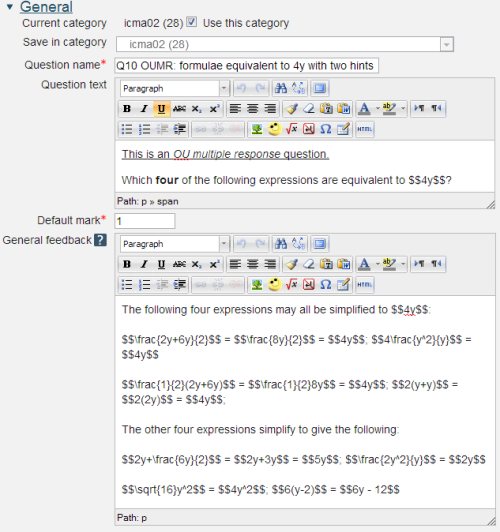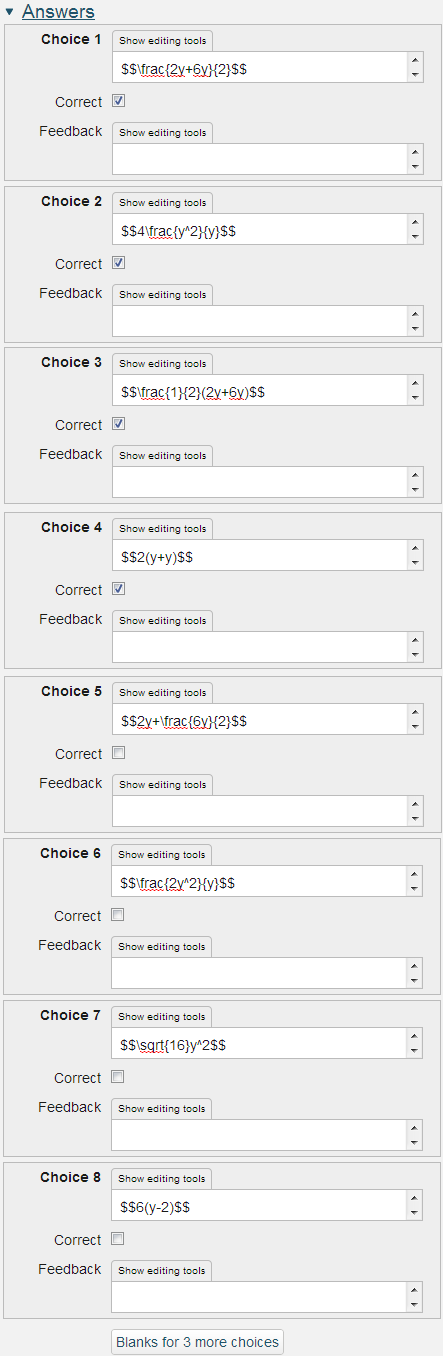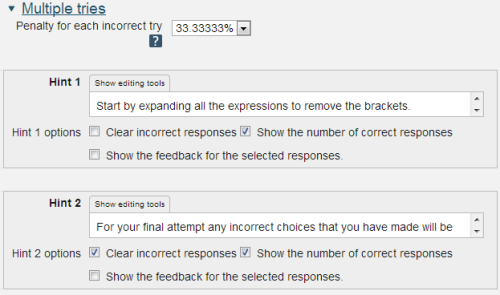# 2.3.5 OU multiple response

Along with the Multiple Choice question type, the Multiple Response question type is the most common form of question used in the OU's paper-based CMA system. The Multiple Response question type requires the student to choose multiple options.2.60 The Question text, mark and General feedback

Question name: A descriptive name is sensible. This name will not be shown to students.

Question text: You may use the full functionality of the editor to state the question.

General feedback: We recommend the correct answer be included in this box. Students who did not answer completely correctly can then compare and contrast to see where they made an error. The contents of this box will be shown to all students irrespective of whether their response was correct or incorrect.2.61 The available choices

The OU Multiple Response question type simplifies the authoring process by incorporating the marking scheme developed for the OU paper-based CMA system. In this marking scheme

• Choices are marked as correct or incorrect. If a question has n correct choices each correct choice is given a mark of (Question grade)/n.
• If a student selects more than n choices the marks are based on the worst n choices.
• It follows that the number of incorrect choices should equal or exceed the number of correct choices (otherwise a student choosing all choices will receive marks).

Example: A question has five choices of which only 'a' and 'b' are correct. How student responses are marked:

• a b: 100% correct
• a c: 50% correct
• a b c: 50% correct - only the two worst choices are marked
• a b c d: 0% correct - only the two worst choices are marked2.62 The Combined feedback section

Whether or not Combined feedback is shown to students is governed by the Specific feedback setting on the iCMA definition form.2.63 The multiple tries section

Penalty for each incorrect try: The available mark is reduced by the penalty for second and subsequent tries. In the example above a correct answer at the second try will score 0.6666667 of the available marks and a correct answer at the third try will score 0.3333334 of the available marks.

If the question is used in interactive with multiple tries mode the marking is modified as follows:

1. At each try only the worst n choices are scored.
2. The mark is reduced for each try by the penalty factor.
3. Allowance is made for when a correct response is first chosen providing it remains chosen in subsequent tries.

Hint: You can complete as many of these boxes as you wish. If you wish to give the student three tries at a question you will need to provide two hints. At runtime when the hints are exhausted the question will finish and the student will be given the general feedback and the question score will be calculated.

Clear incorrect responses: When ‘Try again’ is clicked incorrect choices are cleared.

Show the number of correct responses: Include in the feedback a statement of how many choices are correct.

Show the feedback for the selected responses: This setting controls the feedback to individual choices however if too many choices are made it is suppressed.

2.3.4 Multiple choice

2.3.6 Select missing words